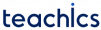# D Flip Flop

The D(data) flip-flop stores the value that is given on the data line. It can be thought of as a basic memory cell.

When both the inputs of an SR flip-flop are at the same logic level, then a no change or invalid output condition occurs. If we avoid these conditions, there will be only two output conditions: SET or RESET. There are many applications, where only SET and RESET conditions of the latch are required. In these applications, we can use inputs (S and R) which are always the complement of each other.

This can be designed by a single input (S) to the latch and the R input achieved by inverting this S. This single input is called data input and it is labelled with D.

### Working of D Flip flop

The circuit diagram of a D flip-flop is given below:

When the clock is set to low, the output remains as it is whether the input signal is set high or low.

When clock is high, if D = 1 then it is equivalent to S = 1 and R = 0 hence the latch is set(1). On the other hand, when D = 0 then it acts like S = 0, and R = 1 hence the latch is reset(0). The characteristics table and truth table of a D flip flop is given below.0 0 0
0 1 1
1 0 0
1 1 1

Characteristics table0 X Qt
1 0 0
1 1 1

Truth table

The equation forand the excitation table derived from the characteristic table is given below :0 0 0
0 1 1
1 0 0
1 1 1